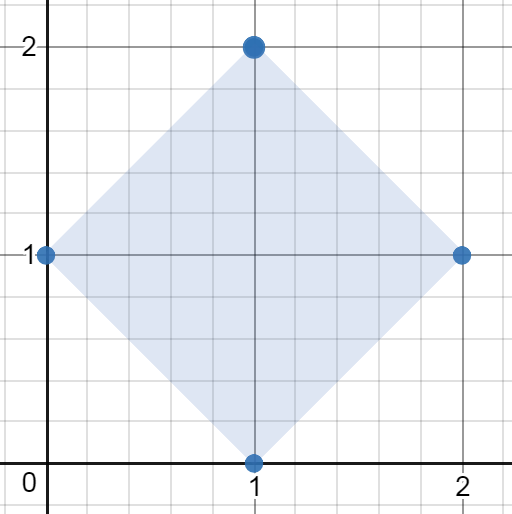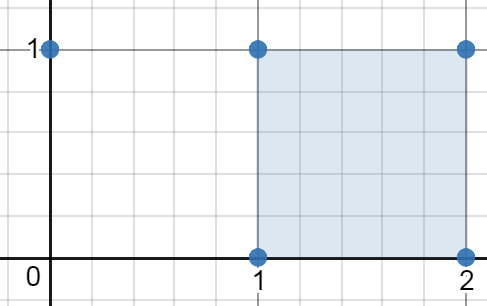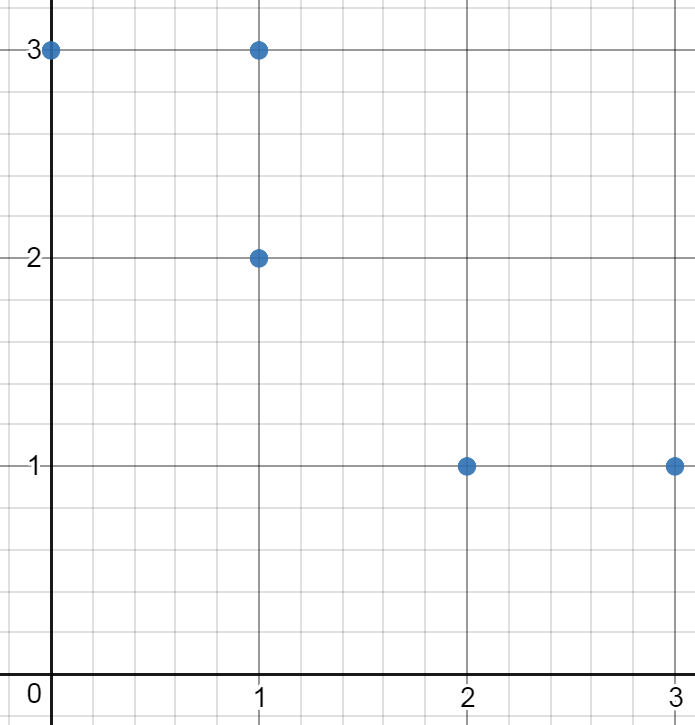## Algorithm

Problem Name: 963. Minimum Area Rectangle II

You are given an array of points in the X-Y plane `points` where `points[i] = [xi, yi]`.

Return the minimum area of any rectangle formed from these points, with sides not necessarily parallel to the X and Y axes. If there is not any such rectangle, return `0`.

Answers within `10-5` of the actual answer will be accepted.

Example 1:```Input: points = [[1,2],[2,1],[1,0],[0,1]]
Output: 2.00000
Explanation: The minimum area rectangle occurs at [1,2],[2,1],[1,0],[0,1], with an area of 2.
```

Example 2:```Input: points = [[0,1],[2,1],[1,1],[1,0],[2,0]]
Output: 1.00000
Explanation: The minimum area rectangle occurs at [1,0],[1,1],[2,1],[2,0], with an area of 1.
```

Example 3:```Input: points = [[0,3],[1,2],[3,1],[1,3],[2,1]]
Output: 0
Explanation: There is no possible rectangle to form from these points.
```

Constraints:

• `1 <= points.length <= 50`
• `points[i].length == 2`
• `0 <= xi, yi <= 4 * 104`
• All the given points are unique.

## Code Examples

### #1 Code Example with Python Programming

```Code - Python Programming```

``````

class Solution:
def minAreaFreeRect(self, points):
mn, st, n = float('inf'), {(x, y) for x, y in points}, len(points)
for i in range(n):
x1, y1 = points[i]
for j in range(i + 1, n):
x2, y2 = points[j]
for k in range(j + 1, n):
x3, y3 = points[k]
if not (x3 - x1) * (x2 - x1) + (y3 - y1) * (y2 - y1) and (x3 + (x2 - x1), y3 + (y2 - y1)) in st:
mn = min(mn, ((x2 - x1) ** 2 + (y2 - y1) ** 2) ** 0.5 * ((x3 - x1) ** 2 + (y3 - y1) ** 2) ** 0.5)
return mn if mn < float("inf") else 0
``````
Copy The Code &

Input

cmd
points = [[1,2],[2,1],[1,0],[0,1]]

Output

cmd
2.00000